# Beer

After three 10° beers consumed in a short time, there is 5.6 g of alcohol in 6 kg adult human blood. How much is it per mille?

Correct result:

p =  0.9

#### Solution: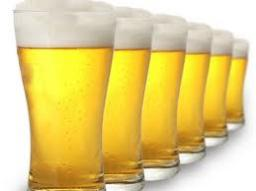We would be pleased if you find an error in the word problem, spelling mistakes, or inaccuracies and send it to us. Thank you!Tips to related online calculators
Need help calculate sum, simplify or multiply fractions? Try our fraction calculator.
Check out our ratio calculator.
Do you have a linear equation or system of equations and looking for its solution? Or do you have quadratic equation?
Do you want to convert mass units?

## Next similar math problems:

• The string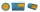They cut 113 cm from the string and divided the rest in a ratio of 5: 6.5: 8: 9.5. The longest part measured 38 cm. Find the original length of the string.
• Fraction to decimal infinite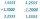Determine which digit is at 1000th place after the decimal point in the decimal expansion of the fraction 9/28.
• Clock mathematicsIf it is now 7:38 pm, what time will it be in 30,033,996,480 minutes from now?
• An airplaneAn airplane flies 1440 km in 2 1/4 hours. What is its average speed in km per hour?
• Milk cratesA farmer sells milk in crates that hold 15 bottles. She has 34,125 bottles of milk. How many crates can the farmer fill?
• An electricianAn electrician needs 1 1/3 rolls of electrical wire to wire each room in a house. How many rooms can he wire with 6 2/3 rolls of wire?
• Borrowing bicycle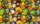Jakub lent a bicycle to friends who wanted to ride it. For a three-hour bike ride, Jakub received 2 chocolates. Yup. If you wanted a bike for 2 hours, you had to give Jakub 12 candies. Peter gave Jakub 1 chocolate and 3 candies. How long can Peter ride Ja
• Drawing from a hatWhen drawing numbers from a hat from 1 to 35, we select random given numbers. What is the probability that the drawn numbers will be divisible by 8 and 2?
• Tennis balls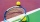Can of tennis balls contains 3 balls per can and cost \$7 how much will it cost for 36 tennis balls?
• Divide in ratioLine segment AB 12 cm long divide in a ratio of 5: 3. How long are the individual parts?
• A farmer's heritage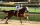A farmer died leaving his 17 horses to his 3 sons. When his sons opened up the Will it read: My eldest son should get 1/2 (half) of total horses; My middle son should be given 1/3rd (one-third) of the total horses; My youngest son should be given 1/9th (o
• The fourThe four pirates divided 65 coins to each other. They were sorted by age, the youngest receiving the least number of coins, each half more than the previous one. How many coins did the oldest pirate receive?
• Father and daughterFather is 36 years old, daughter is 20 years less. What will be the ratio between them when they are 10 years more?
• Two divided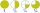Two divided by nine tenths.
• Numbers divisionWith what number should be divided mixed number 2 3/4 to get 11/12?
• A baker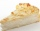A baker has 5 1/4 pies in her shop. She cut the pies in pieces that are each 1/8 of a whole pie. How many pieces of pie does she have?
• Video gameNicole is playing a video game where each round lasts 7/12 of an hour. She has scheduled 3 3/4 hours to play the game. How many rounds can Nicole play?